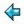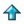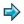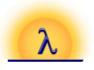### rational.m

```||rational numbers as an abstract data type, say
||      %include <ex/rational>
||to include this in one of your own scripts.  Quoting the  filename  in
||this form makes the %include directive work from any directory.

abstype rational
with ratio :: num -> num -> rational
mkrat :: num->rational
rplus, rminus, rtimes, rdiv :: rational -> rational -> rational
rpow :: num -> rational -> rational
numerator, denominator :: rational -> num
rparts :: rational -> (num,num)
showrational :: rational -> [char]

rational == (num,num)

||a rational is represented as a pair of integers
||note that rationals are kept in their lowest terms, with positive
||denominator, and (0,1) is the unique representation of zero

ratio p q = error "illegal ratio", if  q=0\/~integer p\/~integer q
= ratio (-p) (-q),       if q<0
= (0,1),                 if  p=0
= (p div h,q div h),     otherwise
where
h = hcf (abs p) q
hcf a b = hcf b a,         if  a>b
= b,               if a=0
= hcf (b mod a) a, otherwise

mkrat n = ratio n 1,                   if integer n
= error ("mkrat "++shownum n), otherwise

(a,b) \$rplus (c,d) = ratio (a*d+c*b) (b*d)
(a,b) \$rminus (c,d) = ratio (a*d-c*b) (b*d)
(a,b) \$rtimes (c,d) = ratio (a*c) (b*d)
(a,b) \$rdiv (c,d) = ratio (a*d) (b*c)

rpow 0 x = (1,1)
rpow n x = thing,           if n mod 2 = 0
= x \$rtimes thing, otherwise
where
thing = rpow (n div 2) (x \$rtimes x)

numerator = fst
denominator = snd
rparts = id

showrational (a,b) = "(ratio "++shownum1 a++" "++shownum1 b++")"
shownum1 n = "("++shownum n++")", if n<0
= shownum n,           otherwise

||Attempts to print a rational will automatically pick up  the  function
||called showrational - see manual section on abstract data types.  Note
||that we have chosen to print rationals in such a way that Miranda  can
||read them back in again at the same type.
```